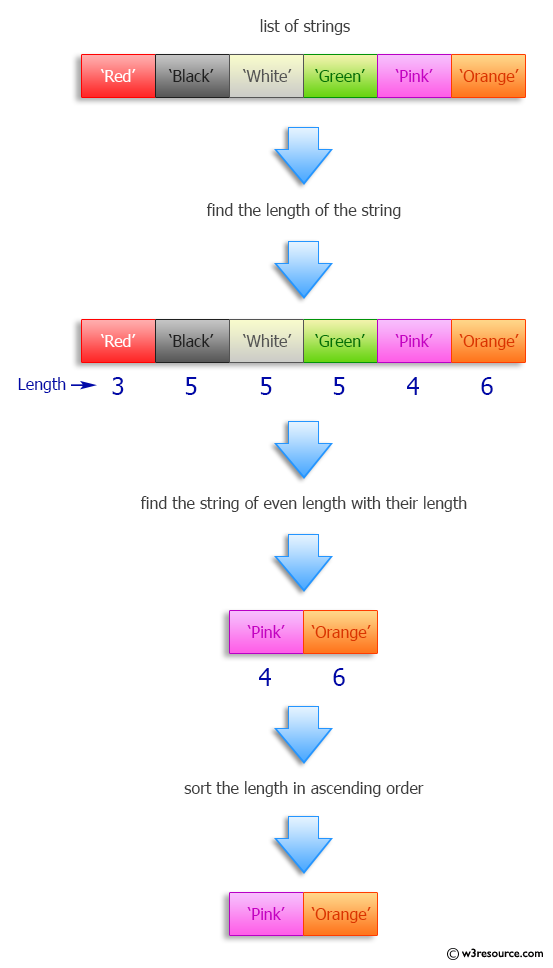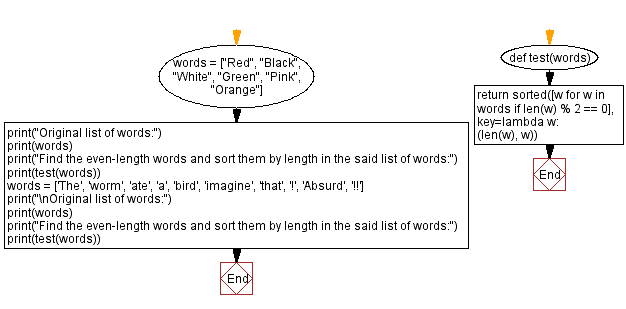﻿ Python: Find the even-length words and sort them by length - w3resource# Python: Find the even-length words and sort them by length

## Python Programming Puzzles: Exercise-50 with Solution

Write a Python program to find the even-length words from a given list of words and sort them by length.

```Input:
['Red', 'Black', 'White', 'Green', 'Pink', 'Orange']
Output:
['Pink', 'Orange']

Input:
['The', 'worm', 'ate', 'a', 'bird', 'imagine', 'that', '!', 'Absurd', '!!']
Output:
['!!', 'bird', 'that', 'worm', 'Absurd']
```

Pictorial Presentation:Sample Solution:

Python Code:

``````#License: https://bit.ly/3oLErEI

def test(nums):
return sorted([w for w in words if len(w) % 2 == 0], key=lambda w: (len(w), w))

words = ["Red", "Black", "White", "Green", "Pink", "Orange"]
print("Original list of words:")
print(words)
print("Find the even-length words and sort them by length in the said list of words:")
print(test(words))
words = ['The', 'worm', 'ate', 'a', 'bird', 'imagine', 'that', '!', 'Absurd', '!!']
print("\nOriginal list of words:")
print(words)
print("Find the even-length words and sort them by length in the said list of words:")
print(test(words))
``````

Sample Output:

```Original list of words:
['Red', 'Black', 'White', 'Green', 'Pink', 'Orange']
Find the even-length words and sort them by length in the said list of words:
['Pink', 'Orange']

Original list of words:
['The', 'worm', 'ate', 'a', 'bird', 'imagine', 'that', '!', 'Absurd', '!!']
Find the even-length words and sort them by length in the said list of words:
['!!', 'bird', 'that', 'worm', 'Absurd']
```

Flowchart:## Visualize Python code execution:

The following tool visualize what the computer is doing step-by-step as it executes the said program:

Python Code Editor :

Have another way to solve this solution? Contribute your code (and comments) through Disqus.

What is the difficulty level of this exercise?

Test your Programming skills with w3resource's quiz.

﻿

## Python: Tips of the Day

Clamps num within the inclusive range specified by the boundary values x and y:

Example:

```def tips_clamp_num(num,x,y):
return max(min(num, max(x, y)), min(x, y))
print(tips_clamp_num(2, 4, 6))
print(tips_clamp_num(1, -1, -6))
```

Output:

```4
-1
```